# Calculus 1 : How to find integral expressions

## Example Questions

1 2 11 12 13 14 15 16 17 19 Next →

### Example Question #181 : Integral Expressions

Solve the following integral, where a and b are constants: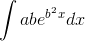Possible Answers:Correct answer:Explanation:

Keeping in mind that a and b are only constants, the integral is equal to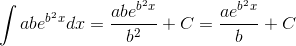and was found using the following rule: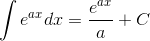### Example Question #181 : How To Find Integral Expressions

Evaluate the following integral: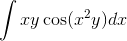Possible Answers: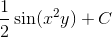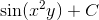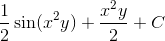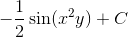Correct answer:Explanation:

To evaluate the integral, we must make the following substitution: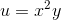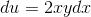The derivative was found using the following rule: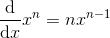Rewrite the integral in terms of u and integrate: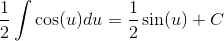The integral was found using the following rule: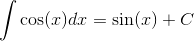Finally, replace u with our original term:.

### Example Question #181 : Integral Expressions

In circuits with a resistor, the equation for voltage drop is given by: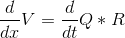, whereis voltage,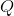is charge, andis resistance.

Write the equation as an integral expression forPossible Answers: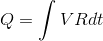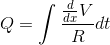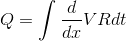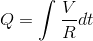Correct answer:Explanation:

Although this may seem really difficult, we only need to solve for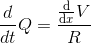To solve for, integrate both sides:### Example Question #1231 : Functions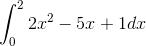Possible Answers: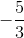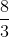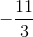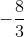Correct answer:Explanation:

When integrating, remember to add one to the exponent and then put that result on the denominator: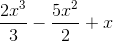. Now evaluate at 2, and then 0. Then subtract the two results.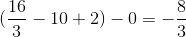.

### Example Question #182 : How To Find Integral Expressions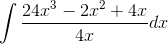Possible Answers: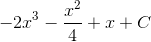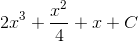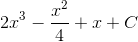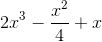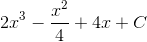Correct answer:Explanation:

The first step here is to chop this up into three separate terms and then simplify since we have only one denominator: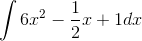. Then, integrate each term, remembering to add one to the exponent and then put that result on the denominator: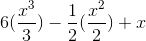. Simplify to get your answer:. Remember to add C because it is an indefinite integral.

### Example Question #186 : Integral Expressions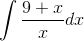Possible Answers:Correct answer:Explanation:

First, chop this expression up into two terms: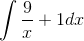. Then, integrate each term, remembering that when there is a single x on a denominator, the integral is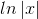. Therefore, the integration is: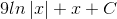. Remember to add C because it is an indefinite integral.

### Example Question #187 : Integral Expressions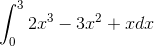Possible Answers:Correct answer:Explanation:

First, integrate each term separately. Remember, when integrating, raise the exponent by one and then also put that result on the denominator: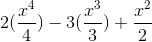. Then evaluate at 3 and then 0. Subtract two results to get: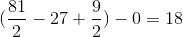.

### Example Question #188 : Integral Expressions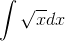Possible Answers:Correct answer:Explanation:

To integrate this expression, I would first rewrite it to be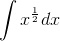. Then, add one to the exponent and then put that result on the denominator: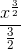. Then, simplify to get your answer of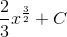. Remember to add C because it is an indefinite integral.

1 2 11 12 13 14 15 16 17 19 Next →

### All Calculus 1 Resources